## More circular motion

10-1-99

Sections 5.3 - 5.5

### Cars on banked turns

A good example of uniform circular motion is a car going around a banked turn, such as on a highway off-ramp. These off-ramps often have the recommended speed posted; even if there was no friction between your car tires and the road, if you went around the curve at this "design speed" you would be fine. With no friction, if you went faster than the design speed you would veer towards the outside of the curve, and if you went slower than the design speed you would veer towards the inside of the curve.

In theory, then, accounting for friction, there is a range of speeds at which you can negotiate a curve. In most cases the coefficient of friction is sufficiently high, and the angle of the curve sufficiently small, that going too slowly around the curve is not an issue. Going too fast is another story, however.

The textbook does a good analysis of a car on a banked curve without friction, arriving at a connection between the angle of the curve, the radius, and the speed. The speed is known as the design speed of the curve (the speed at which you're safest negotiating the curve) and is given by: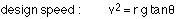Consider now the role that friction plays, and think about how to determine the maximum speed at which you can negotiate the curve without skidding. The first thing to realize is that the frictional force is static friction. Even though the car is moving, the car tires are not slipping on the road surface, so the part of tire in contact with the road is instantaneously at rest with respect to the road. Also, if we're worried about the maximum speed at which we can go around the banked turn, if there was no friction the car would tend to slide towards the outside of the curve, so the friction opposes this tendency and points down the slope.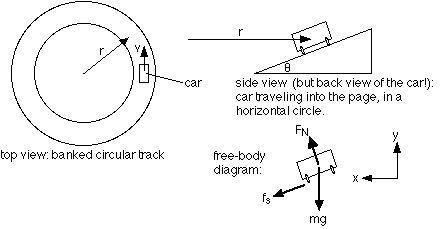The diagram, and a free-body diagram, of the situation is shown here. Note that the diagram looks similar to that of a box on an inclined plane. There is a critical difference, however; for the box on an inclined plane, the coordinate system was parallel and perpendicular to the slope, because the box was either moving, and/or accelerating, up or down the slope. In this case the coordinate system is horizontal and vertical, because the centripetal acceleration points horizontally in towards the center of the circle and there is no vertical component of the acceleration.

Moving from the free-body diagram to the force equations gives: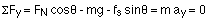This can be rearranged to solve for the normal force: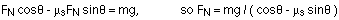Note that we're solving for the maximum speed at which the car can go around the curve, which will correspond to the static force of friction being a maximum, which is why it's valid to say that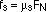.

In the x-direction, the force equation is: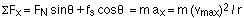Substituting, and plugging in the equation for the normal force, gives: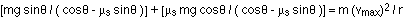There is an m in every term, so the mass cancels out. The equation can then be rearranged to solve for the maximum speed: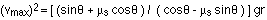Note that when the coefficient of friction is zero (i.e., the road is very slippery), the maximum speed reduces to the design speed,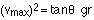and for certain combinations of theta and the coefficient of friction (both large, in general) the denominator turns out to be negative, implying that there is no maximum speed; in those cases, you could drive as fast as you wanted without worrying about skidding. Note that this does not apply to standard highway off-ramps! The appropriate conditions for no maximum safe speed (or at least a very high maximum) would be found at racetracks like the Indianapolis Speedway, for example.

### Vertical circular motion

Some roller-coasters have loop-the-loop sections, where you travel in a vertical circle, so that you're upside-down at the top. To do this without falling off the track, you have to be traveling at least a particular minimum speed at the top. The critical factor in determining whether you make it completely around is the normal force; if the track has to exert a downward normal force at the top of the track to keep you moving in a circle, you're fine, but if the normal force drops to zero you're in trouble.

The normal force changes as you travel around a vertical loop; it changes because your speed changes and because your weight has a different effect at each part of the circle. To keep going in a circular path, you must always have a net force equal to mv2 / r pointing towards the center of the circle. If the net force drops below the required value, you will veer off the circular path away from the center, and if the net force is more than the required value you will veer off towards the center.

Consider what happens at the bottom of the loop, and compare it to what happens at the top. At the bottom, mg points down and the normal force points up, towards the center of the circle. The normal force is then not simply equal to the weight, but is larger because it must also supply the required centripetal acceleration: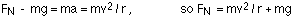This is why you actually feel heavier at the bottom of a loop like this, because your apparent weight is equal to the normal force you feel.

At the top of the loop, on the other hand, the normal force and the weight both point towards the center of the circle, so the normal force is less than the weight:If you're going at just the right speed so mv2 / r = mg, the normal force drops to zero, and you would actually feel weightless for an instant. Faster than this speed and there is a normal force helping to keep you on the circular path; slower and the normal force would go up, which means you'll fall out of the coaster and/or the coaster will fall off the track unless you're strapped in and the coaster is held down to the track.